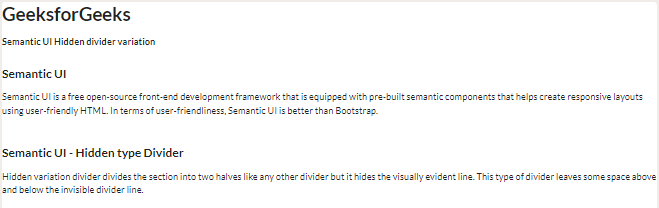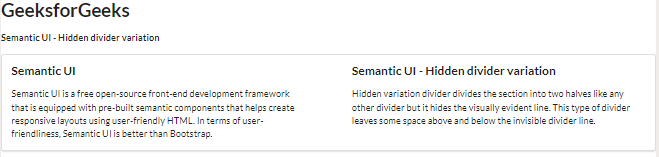# Semantic-UI Divider Variations Hidden Variant

• Last Updated : 02 Feb, 2022

Semantic UI is a free open-source front-end development framework that is equipped with pre-built semantic components that helps create responsive layouts using user-friendly HTML.  It uses predefined CSS and jQuery to incorporate different frameworks.

A Semantic UI divider is generally used to divide the content concisely into different sections. Semantic UI has 3 different types of dividers, each type can have 5 different variations. In this article, let us discuss hidden variations in the divider variations supported in Semantic UI.

A Semantic UI hidden divider divides the webpage content without creating a dividing line, unlike other variations. Hidden variation divider divides the section into two halves like any other divider but it hides the visually evident line. This type of divider leaves some space above and below the invisible divider line.

Semantic-UI Divider Variations Hidden Variant Class:

• hidden:

Syntax:

```<h3 class="ui header">Section One</h3>
<div class="ui hidden divider"></div>

Below example illustrate the Semantic-UI Divider Variations Hidden Variant:

Example 1: This example hides the horizontal divider using hidden divider class.

## HTML

 ` ` `<``html``> ` ` `  `<``head``> ` `    ``<``link` `href``= ` `"https://cdnjs.cloudflare.com/ajax/libs/semantic-ui/2.4.1/semantic.min.css"`  `          ``rel``=``"stylesheet"` `/> ` ` `  `<``body``> ` `    ``<``h1``>GeeksforGeeks  ` `    ``<``strong``>Semantic UI Hidden divider variation ` `    ``<``h3` `class``=``"ui header"``> Semantic UI ` `    ``<``p``> ` `        ``Semantic UI is a free open-source front-end  ` `        ``development framework that is equipped with ` `        ``pre-built semantic components that helps create  ` `        ``responsive layouts using user-friendly HTML. In  ` `        ``terms of user-friendliness, Semantic UI is better ` `        ``than Bootstrap. ` `    `` ` ` `  `    ``<``div` `class``=``"ui hidden divider"``> ` `    ``<``h3` `class``=``"ui header"``>  ` `        ``Semantic UI - Hidden type Divider  ` `    `` ` `    ``<``p``> ` `        ``Hidden variation divider divides the section ` `        ``into two halves like any other divider but it ` `        ``hides the visually evident line. This type of ` `        ``divider leaves some space above and below the ` `        ``invisible divider line.  ` `    `` ` ` `  ` ` ` `  ``

Output:Semantic-UI Divider Variations Hidden Variant

Example 2: In the below example, we can see a hidden divider variation using a vertical divider, which divides two sections into inverted vertical sections.

## HTML

 ` ` `<``html``> ` ` `  `<``head``> ` `    ``<``link` `href``= ` `"https://cdnjs.cloudflare.com/ajax/libs/semantic-ui/2.4.1/semantic.min.css"`  `          ``rel``=``"stylesheet"` `/> ` ` ` ` `  `<``body``> ` `    ``<``h1``>GeeksforGeeks ` `    ``<``strong``> Semantic UI - Hidden divider variation ` `    ``<``div` `class``=``"ui segment"``> ` `        ``<``div` `class``=``"ui two column very relaxed grid"``> ` `            ``<``div` `class``=``"column"``> ` `                ``<``h3` `class``=``"ui header"``> Semantic UI ` `                ``<``p``> ` `                    ``Semantic UI is a free open-source front-end ` `                    ``development framework that is equipped with  ` `                    ``pre-built semantic components that helps create ` `                    ``responsive layouts using user-friendly HTML.  ` `                    ``In terms of user-friendliness, Semantic UI is ` `                    ``better than Bootstrap. ` `                `` ` ` `  `            `` ` `            ``<``div` `class``=``"column"``> ` `                ``<``h3` `class``=``"ui header"``>  ` `                    ``Semantic UI - Hidden divider variation  ` `                `` ` `                ``<``p``> ` `                    ``Hidden variation divider divides the section  ` `                    ``into two halves like any other divider but it ` `                    ``hides the visually evident line. This type of ` `                    ``divider leaves some space above and below the ` `                    ``invisible divider line. ` `                `` ` ` `  `            `` ` `        `` ` `        ``<``div` `class``=``"ui hidden vertical divider"``> ` `    `` ` ` ` ` `  ``

Output:Semantic-UI Divider Variations Hidden Variant

My Personal Notes arrow_drop_up
Recommended Articles
Page :